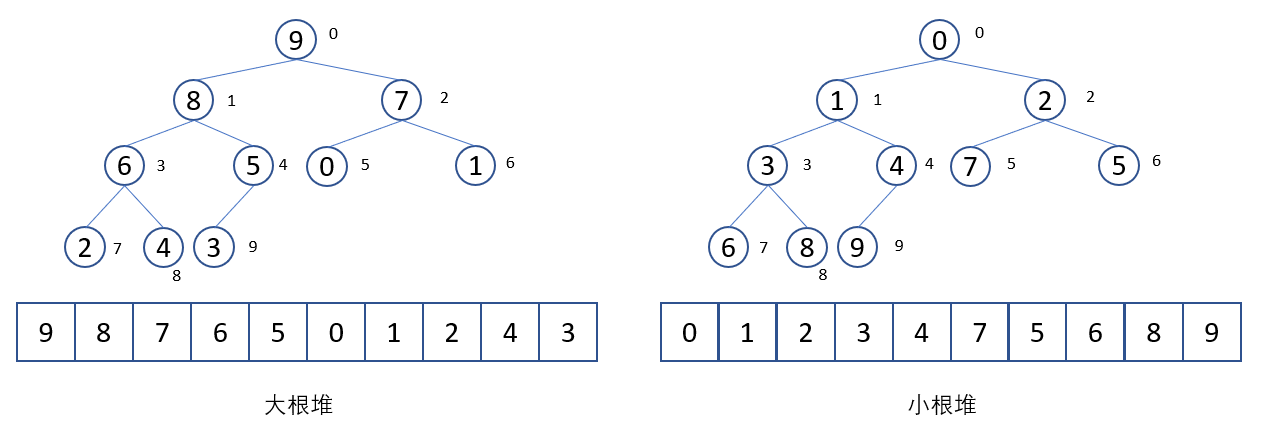# 一、优先队列• 第$i$个节点的左孩子的下标为$2i+1$，右孩子的下标为$2i+2$。
• 第$i$个节点的父亲节点的下标为$(i-1)//2$

• 插入操作：$O(\log n)$
• 删除操作：$O(\log n)$
• 查询最小值：$O(1)$

python实现：heapq

import heapq

# 将array列表转为堆的结构
heap.heapify(array)
# 弹出堆中的最小值
heapq.heappop(array)
# 往堆中插入新值a
heapq.heappush(array, a)
# 先进行heappop(array)，再进行heappush(array, a)操作
heapq.heapreplace(array, a)
# 获得array中前k个最大的值
heapq.nlargest(k, array)
# 获得array中前k个最小的值
heapq.nsmallest(k, array)

# 二、例题

### 1、合并k个有序链表

import heapq
class Solution:
def mergeKLists(self, lists: List[ListNode]) -> ListNode:
# 建立堆
a = []
for i in range(len(lists)):
if lists[i] :
# 元组在heapq里比较的机制是从元组首位0开始，即遇到相同，就比较元组下一位
# 比如(1,2), (1,3)，前者比后者小。
# 这题刚好node值有重复的，同时ListNode无法被比较，所以会报错
heapq.heappush(a, (lists[i].val, i))
lists[i] = lists[i].next
while a:
val, idx = heapq.heappop(a)
p.next = ListNode(val)
p = p.next
if lists[idx]:
heapq.heappush(a, (lists[idx].val, idx))
lists[idx] = lists[idx].next
return head.next
• 时间复杂度：$O(n \log k)$
• 空间复杂度：$O(k+n)$，最小堆需要k个空间，新链表需要n个空间

### 2、数据流中的第k大元素

import heapq
class KthLargest:
def __init__(self, k: int, nums: List[int]):
self.nums = nums
self.k = k
heapq.heapify(self.nums)
# 留下k个元素，即前k大的
while len(self.nums) > k:
heapq.heappop(self.nums)

def add(self, val: int) -> int:
if len(self.nums) < self.k:
heapq.heappush(self.nums, val)
elif self.nums < val:
# 新的值更大则更新
heapq.heapreplace(self.nums, val)

return self.nums